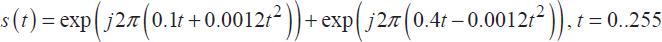## 12.3. Exercises

EXERCISE 12.11.

Calculate the FRFT to the sum of two chirps given below:for an order sweeping the range between 0 and 2 with an increment of 0.1.

1. Plot the instantaneous frequency law for each component of the signal.
2. Estimate the linear modulation rate using different FRFT representations.

EXERCISE 12.12.

Consider the signal:where b stands for a Gaussian noise.

1. Estimate the signal parameters using the FRFT.
2. Calculate the minimum SNR, for which it is still possible to estimate these parameters.

EXERCISE 12.13.

Consider the following signal:Express the time-frequency content of this signal as the sum of two chirps using the FRFT.

EXERCISE 12.14.

Consider the signal below:1. Plot the instantaneous frequency law for each component of the signal.
2. Estimate the parameters of each component using the FRFT.
3. Propose a solution to increase the obtained resolution.

EXERCISE 12.15.

Let us consider the following sinusoidal frequency modulation:1. Model the phase of this signal ...

Get Digital Signal Processing Using Matlab now with the O’Reilly learning platform.

O’Reilly members experience live online training, plus books, videos, and digital content from nearly 200 publishers.